Related Articles

# PySpark Collect() – Retrieve data from DataFrame

• Last Updated : 17 Jun, 2021

Collect() is the function, operation for RDD or Dataframe that is used to retrieve the data from the Dataframe. It is used useful in retrieving all the elements of the row from each partition in an RDD and brings that over the driver node/program.

So, in this article, we are going to learn how to retrieve the data from the Dataframe using collect() action operation.

Syntax: df.collect()

Where df is the dataframe

Example 1: Retrieving all the Data from the Dataframe using collect().

After creating the Dataframe, for retrieving all the data from the dataframe we have used the collect() action by writing df.collect(), this will return the Array of row type, in the below output shows the schema of the dataframe and the actual created Dataframe.

## Python

 `# importing necessary libraries``from` `pyspark.sql ``import` `SparkSession`` ` `# function to create new SparkSession``def` `create_session():``  ``spk ``=` `SparkSession.builder \``      ``.appName(``"Corona_cases_statewise.com"``) \``      ``.getOrCreate()``  ``return` `spk`` ` `# function to create RDD``def` `create_RDD(sc_obj,data):``  ``df ``=` `sc.parallelize(data)``  ``return` `df`` ` ` ` `if` `__name__ ``=``=` `"__main__"``:``     ` `  ``input_data ``=` `[(``"Uttar Pradesh"``,``122000``,``89600``,``12238``),``          ``(``"Maharashtra"``,``454000``,``380000``,``67985``),``          ``(``"Tamil Nadu"``,``115000``,``102000``,``13933``),``          ``(``"Karnataka"``,``147000``,``111000``,``15306``),``          ``(``"Kerala"``,``153000``,``124000``,``5259``)]`` ` `  ``# calling function to create SparkSession``  ``spark ``=` `create_session()`` ` `  ``# creating spark context object``  ``sc ``=` `spark.sparkContext`` ` `  ``# calling function to create RDD``  ``rd_df ``=` `create_RDD(sc,input_data)`` ` `  ``schema_lst ``=` `[``"State"``,``"Cases"``,``"Recovered"``,``"Deaths"``]`` ` `  ``# creating the dataframe using createDataFrame function``  ``df ``=` `spark.createDataFrame(rd_df,schema_lst)``  ``# printing schema of the dataframe and showing the dataframe``  ``df.printSchema()``  ``df.show()``     ` `  ``# retrieving the data from the dataframe using collect() ``  ``df2``=` `df.collect()``  ``print``(``"Retrieved Data is:-"``)``  ``print``(df2)  `

Output: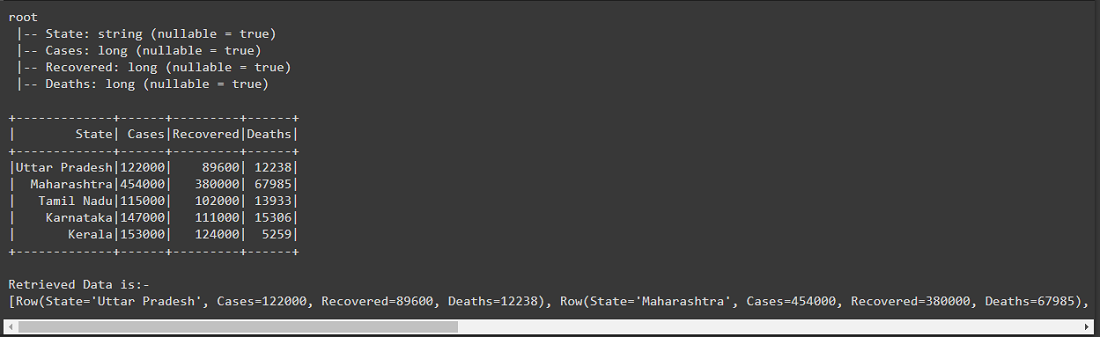Example 2: Retrieving Data of specific rows using collect().

After creating the Dataframe, we have retrieved the data of 0th row Dataframe using collect() action by writing print(df.collect()[0:]) respectively in this we are passing row and column after collect(), in the first print statement we have passed row and column as [0:] here first  represents the row that we have passed 0 and second [0:] this represents the column and colon(:) is used to retrieve all the columns, in short, we have retrieve the 0th row with all the column elements.

## Python

 `# importing necessary libraries``from` `pyspark.sql ``import` `SparkSession`` ` `# function to create new SparkSession``def` `create_session():``  ``spk ``=` `SparkSession.builder \``      ``.appName(``"Corona_cases_statewise.com"``) \``      ``.getOrCreate()``  ``return` `spk`` ` `# function to create RDD``def` `create_RDD(sc_obj,data):``  ``df ``=` `sc.parallelize(data)``  ``return` `df`` ` ` ` `if` `__name__ ``=``=` `"__main__"``:``     ` `  ``input_data ``=` `[(``"Uttar Pradesh"``,``122000``,``89600``,``12238``),``          ``(``"Maharashtra"``,``454000``,``380000``,``67985``),``          ``(``"Tamil Nadu"``,``115000``,``102000``,``13933``),``          ``(``"Karnataka"``,``147000``,``111000``,``15306``),``          ``(``"Kerala"``,``153000``,``124000``,``5259``)]`` ` `  ``# calling function to create SparkSession``  ``spark ``=` `create_session()`` ` `  ``# creating spark context object``  ``sc ``=` `spark.sparkContext`` ` `  ``# calling function to create RDD``  ``rd_df ``=` `create_RDD(sc,input_data)`` ` `  ``schema_lst ``=` `[``"State"``,``"Cases"``,``"Recovered"``,``"Deaths"``]`` ` `  ``# creating the dataframe using createDataFrame function``  ``df ``=` `spark.createDataFrame(rd_df,schema_lst)`` ` `  ``# printing schema of the dataframe and showing the dataframe``  ``df.printSchema()``  ``df.show()``   ` `  ``print``(``"Retrieved Data is:-"``)``   ` `  ``# Retrieving data from 0th row ``  ``print``(df.collect()[``0``][``0``:])`

Output: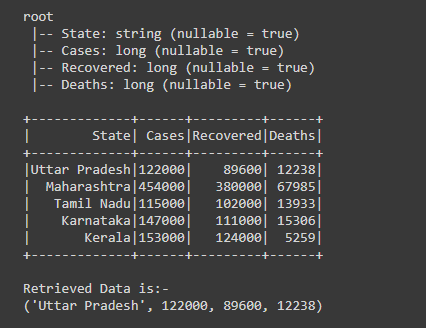Example 3: Retrieve data of multiple rows using collect().

After creating the Dataframe, we are retrieving the data of the first three rows of the dataframe using collect() action with for loop, by writing for row in df.collect()[0:3], after writing the collect() action we are passing the number rows we want [0:3], first  represents the starting row and using “:” semicolon and  represents the ending row till which we want the data of multiple rows.

Here is the number of rows from which we are retrieving the data is 0,1 and 2 the last index is always excluded i.e, 3.

## Python

 `# importing necessary libraries``from` `pyspark.sql ``import` `SparkSession``from` `pyspark.sql.functions ``import` `col`` ` `# function to create new SparkSession``def` `create_session():``  ``spk ``=` `SparkSession.builder \``      ``.appName(``"Corona_cases_statewise.com"``) \``      ``.getOrCreate()``  ``return` `spk`` ` `# function to create RDD``def` `create_RDD(sc_obj,data):``  ``df ``=` `sc.parallelize(data)``  ``return` `df`` ` ` ` `if` `__name__ ``=``=` `"__main__"``:``     ` `  ``input_data ``=` `[(``"Uttar Pradesh"``,``122000``,``89600``,``12238``),``          ``(``"Maharashtra"``,``454000``,``380000``,``67985``),``          ``(``"Tamil Nadu"``,``115000``,``102000``,``13933``),``          ``(``"Karnataka"``,``147000``,``111000``,``15306``),``          ``(``"Kerala"``,``153000``,``124000``,``5259``)]`` ` `  ``# calling function to create SparkSession``  ``spark ``=` `create_session()`` ` `  ``# creating spark context object``  ``sc ``=` `spark.sparkContext`` ` `  ``# calling function to create RDD``  ``rd_df ``=` `create_RDD(sc,input_data)`` ` `  ``schema_lst ``=` `[``"State"``,``"Cases"``,``"Recovered"``,``"Deaths"``]`` ` `  ``# creating the dataframe using createDataFrame function``  ``df ``=` `spark.createDataFrame(rd_df,schema_lst)`` ` `  ``# showing the dataframe and schema``  ``df.printSchema()``  ``df.show()``   ` `  ``print``(``"Retrieved Data is:-"``)``   ` `  ``# Retrieving multiple rows using collect() and for loop``  ``for` `row ``in` `df.collect()[``0``:``3``]:``    ``print``((row[``"State"``]),``","``,``str``(row[``"Cases"``]),``","``,``          ``str``(row[``"Recovered"``]),``","``,``str``(row[``"Deaths"``]))`

Output: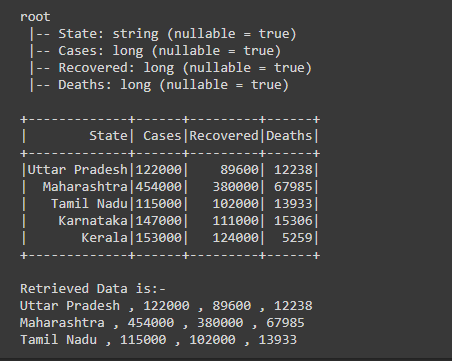Example 4: Retrieve data from a specific column using collect().

After creating the Dataframe, we are retrieving the data of ‘Cases’ column using collect() action with for loop. By iterating the loop to df.collect(), that gives us the Array of rows from that rows we are retrieving and printing the data of ‘Cases’ column by writing print(col[“Cases”]);

As we are getting the rows one by iterating for loop from Array of rows, from that row we are retrieving the data of “Cases” column only. By  writing print(col[“Cases”]) here from each row we are retrieving the data of ‘Cases’ column by passing ‘Cases’ in col.

## Python

 `# importing necessary libraries``from` `pyspark.sql ``import` `SparkSession``from` `pyspark.sql.functions ``import` `col`` ` `# function to create new SparkSession``def` `create_session():``  ``spk ``=` `SparkSession.builder \``      ``.appName(``"Corona_cases_statewise.com"``) \``      ``.getOrCreate()``  ``return` `spk`` ` `# function to create RDD``def` `create_RDD(sc_obj,data):``  ``df ``=` `sc.parallelize(data)``  ``return` `df`` ` `if` `__name__ ``=``=` `"__main__"``:``     ` `  ``input_data ``=` `[(``"Uttar Pradesh"``,``122000``,``89600``,``12238``),``          ``(``"Maharashtra"``,``454000``,``380000``,``67985``),``          ``(``"Tamil Nadu"``,``115000``,``102000``,``13933``),``          ``(``"Karnataka"``,``147000``,``111000``,``15306``),``          ``(``"Kerala"``,``153000``,``124000``,``5259``)]`` ` `  ``# calling function to create SparkSession``  ``spark ``=` `create_session()`` ` `  ``# creating spark context object``  ``sc ``=` `spark.sparkContext`` ` `  ``# calling function to create RDD``  ``rd_df ``=` `create_RDD(sc,input_data)`` ` `  ``schema_lst ``=` `[``"State"``,``"Cases"``,``"Recovered"``,``"Deaths"``]`` ` `  ``# creating the dataframe using createDataFrame function``  ``df ``=` `spark.createDataFrame(rd_df,schema_lst)`` ` `  ``# showing the dataframe and schema``  ``df.printSchema()``  ``df.show()``   ` `  ``print``(``"Retrieved Data is:-"``)``   ` `  ``# Retrieving data from the "Cases" column``  ``for` `col ``in` `df.collect():``    ``print``(col[``"Cases"``])`

Output: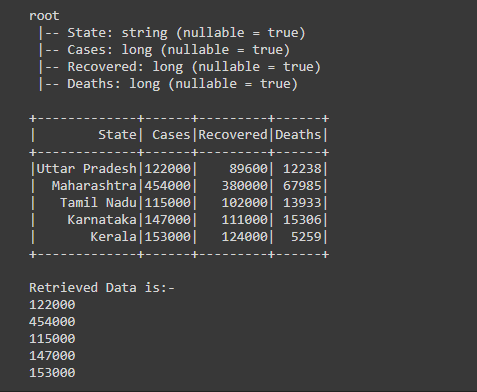Example 5: Retrieving the data from multiple columns using collect().

After creating the dataframe, we are retrieving the data of multiple columns which include “State”, “Recovered” and “Deaths”.

For retrieving the data of multiple columns, firstly we have to get the Array of rows which we get using df.collect() action now iterate the for loop of every row of Array, as by iterating we are getting rows one by one so from that row we are retrieving the data of “State”, “Recovered” and “Deaths” column from every column and printing the data by writing, print(col[“State”],”,”,col[“Recovered”],”,”,col[“Deaths”])

## Python

 `# importing necessary libraries``from` `pyspark.sql ``import` `SparkSession``from` `pyspark.sql.functions ``import` `col`` ` `# function to create new SparkSession``def` `create_session():``  ``spk ``=` `SparkSession.builder \``      ``.appName(``"Corona_cases_statewise.com"``) \``      ``.getOrCreate()``  ``return` `spk`` ` `# function to create RDD``def` `create_RDD(sc_obj,data):``  ``df ``=` `sc.parallelize(data)``  ``return` `df`` ` ` ` `if` `__name__ ``=``=` `"__main__"``:``     ` `  ``input_data ``=` `[(``"Uttar Pradesh"``,``122000``,``89600``,``12238``),``          ``(``"Maharashtra"``,``454000``,``380000``,``67985``),``          ``(``"Tamil Nadu"``,``115000``,``102000``,``13933``),``          ``(``"Karnataka"``,``147000``,``111000``,``15306``),``          ``(``"Kerala"``,``153000``,``124000``,``5259``)]`` ` `  ``# calling function to create SparkSession``  ``spark ``=` `create_session()`` ` `  ``# creating spark context object``  ``sc ``=` `spark.sparkContext`` ` `  ``# calling function to create RDD``  ``rd_df ``=` `create_RDD(sc,input_data)`` ` `  ``schema_lst ``=` `[``"State"``,``"Cases"``,``"Recovered"``,``"Deaths"``]`` ` `  ``# creating the dataframe using createDataFrame function``  ``df ``=` `spark.createDataFrame(rd_df,schema_lst)`` ` `  ``# showing the dataframe and schema``  ``df.printSchema()``  ``df.show()``   ` `  ``print``(``"Retrieved Data is:-"``)``   ` `  ``# Retrieving data of the "State",``  ``# "Recovered" and "Deaths" column``  ``for` `col ``in` `df.collect():``    ``print``(col[``"State"``],``","``,col[``"Recovered"``],",``          ``",col["``Deaths"])`

Output: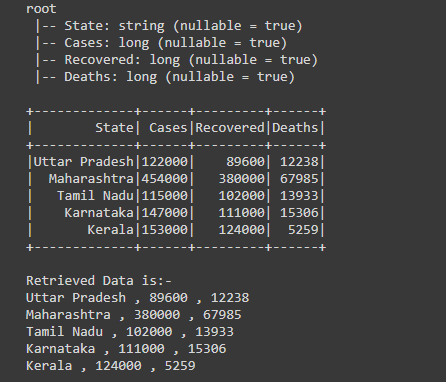Attention geek! Strengthen your foundations with the Python Programming Foundation Course and learn the basics.

To begin with, your interview preparations Enhance your Data Structures concepts with the Python DS Course. And to begin with your Machine Learning Journey, join the Machine Learning – Basic Level Course

My Personal Notes arrow_drop_up